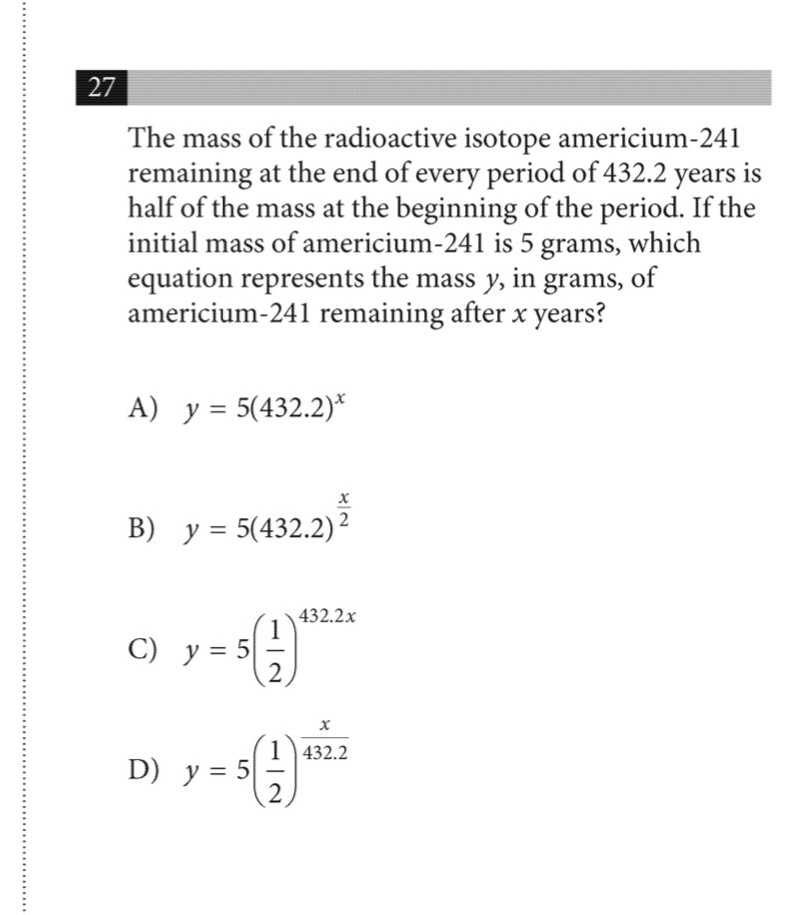### Still have math questions?

Algebra
QuestionThe mass of the radioactive isotope americium- $$241$$ remaining at the end of every period of $$432.2$$ years is half of the mass at the beginning of the period. If the initial mass of americium- $$241$$ is $$5$$ grams, which equation represents the mass $$y$$ , in grams, of americium-241 remaining after $$x$$ years?

A) $$y = 5 ( 432.2 ) ^ { x }$$

B) $$y = 5 ( 432.2 ) ^ { \frac { x } { 2 } }$$

C) $$y = 5 ( \frac { 1 } { 2 } ) ^ { 432.2 x }$$

D) $$y = 5 ( \frac { 1 } { 2 } ) ^ { \frac { x } { 432.2 } }$$